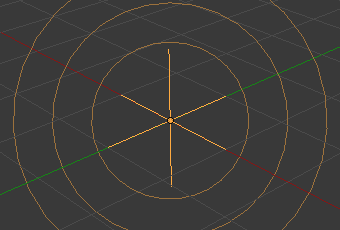# Introduction¶

Force fields offer a way to influence a simulation, in example to add extra movement. Particles, Soft Bodies, Rigid Bodies, and Cloth objects can all be affected by forces fields. Force fields automatically affect everything. To remove a simulation or particle system from their influence, simply turn down the influence of that type of force field in its Field Weights panel.

• All types of objects and particles can generate fields, but only curve object can bear a Curve Guide field.

• Force fields can also be generated from particles. See Particle Physics.

• The objects need to share at least one common layer to have an effect.

You may limit the effect on particles to a group of objects (see the Particle Physics page).

## Creating a Force Field¶

Reference

Mode

Object Mode

Panel

Physics ‣ Force Field

To create a single force field, you can select Add ‣ Force Field and select the desired force field. This method creates an empty with the force field attached.Vortex force field.¶Wind force field.¶Force force field.¶

To create a field from an existing object you have to select the object and change to the Physics tab. Select the field type in the Fields menu.

Note

After changing the fields Fields panel or deflection Collision panel settings, you have to recalculate the particle, soft body or cloth system by Free Cache, this is not done automatically.

Particles react to all kinds of force fields, soft bodies only to Force, Wind, Vortex (they react on Harmonic fields but not in a useful way).

## Common Field Settings¶

Most fields have the same settings, even though they act very differently. Settings unique to a field type are described below. Curve Guide and Texture fields have very different options.

Shape

Sets the direction which is used to calculate the effector force. For force fields from an empty object only Point, Line and Plane shapes are available, as for a field from a 3D object there are additional Surface and Every Point options, and Curve for a field from a curve.

Point

Point with omni-directional influence. Uses the object origin as the effector point.

Line

The force only acts in the local XY plane, using the Z axis line as the effector.

Plane

The force only acts in the local Z direction, using the XY axis plane as the effector.

Surface

The force field acts on a 3D object’s surface. In this case, the Z axis is the surface normal.

Every Point

Uses every vertex in the mesh object as an effector point.

Curve

The force field acts along a curve object.

Strength

The strength of the field effect. This can be positive or negative to change the direction that the force operates in. A force field’s strength is scaled with the force object’s scale, allowing you to scale up and down the scene, keeping the same effects.

Flow

If non-zero, this adds a drag force proportional and opposite to the point velocity.

This effectively re-interprets the force field so that the Strength to Flow ratio at a certain point defines the velocity of an “air flow” field, and objects are encouraged to follow the flow by the resistance caused by the Flow drag force.

Noise Amount

Adds noise to the strength of the force.

Seed

Changes the seed of the random noise.

Affect
Location

Influence the location of particles and other physics entities.

Rotation

Influence the rotation of particles with Dynamic Rotation. The option is not relevant for other types of physics systems.

Disabling both options completely deactivates the force field.

Absorption

Force gets absorbed by collision objects.

Wind Factor

Specifies how much the force is reduced when acting parallel to a surface, e.g. cloth. If set to 1, only the normal component of the force is taken into account.

### Falloff¶

Here you can specify the shape of the force field (if the falloff Power is greater than 0).

Shape
Sphere

The falloff is uniform in all directions, as in a sphere.

Tube

The falloff results in a tube-shaped force field. The field’s Radial Power can be adjusted, as well as the Minimum and Maximum distances of the field.

Cone

The falloff results in a cone-shaped force field. Additional options are the same as those of Tube options.

Z Direction

The force can be set to apply only in the direction of the positive Z axis, negative Z axis, or both.

Power

How the power of the force field changes with the distance from the force field. If r is the distance from the origin of the object, the force changes with 1/(r - min + 1)power. A falloff of 2 changes the force field with 1/(r - min + 1)2, which is similar to the falloff of gravitational pull.

Min Distance

The distance from the object’s origin, up to where the force field is effective with full strength. If you have a falloff of 0, this parameter will have no effect, because the field is effective with full strength up to Max Distance (or infinite). Shown by an additional circle around the object.

Max Distance

Specifies the maximum radius in which the force field affects other objects (shown by an additional circle around the object).